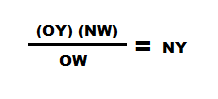### How Much Yarn?

Recently I helped a student calculate the amount of yarn she had leftover. She had worked a scarf from two balls of yarn and had no idea how much yarn was used and was hoping to have enough for a matching hat. I told her it would be no problem to calculate approximate yarn amounts based on the weight and I was met by others in the class looking confused or with wonder.

So here's a quick little tutorial on how I calculated the approximate left over yardage.

Materials Needed:

• Left over yarn
• Yarn information (weight and amounts started with)
• Basic Kitchen scale
• Calculator (because although I love math, I can't do calculations in my head, no matter how easy!)

Procedure:

Start with your left-over yarn. If you have the original ball band that is great, you can use the numbers from there. If you don't have the ball band but you know the name of the yarn, manufaturer or anything that can be used to figure out the yarn specs plug it into Ravelry (first shot), Yarndex (second shot) or Google (third shot). Hopefully you can find the original amounts (weight and yardage/meters) the skein was.

In this situation we were lucky and we had the ball band:

So we started with approximately (notice this approx!) 138 yards (126 meters) and 3.5 oz (100 grams).

I typically work in metrics -- except (for some reason) when I talk about yards of yarn. Very odd, I know. But here I'll show the calculations for both metric and US system.

Next step is to weigh the yarn. If you have a basic kitchen scale you can do this.

When you turn it on make sure you notice if it is in grams or oz. Typically (depending on the scale) you can change to your preferred system by pushing a "units" button.

Next you will need to make sure to "tare" the scale so it reads "0". If you find that your yarn does not sit properly on the scale put a light bowl (or another container) onto the scale and hit "tare." Now we have the scale set to 0.

Now is the easy part. Put your skein on the scale and see how much your leftover yarn weighs.

Here you can see we have 95 grams. If we wanted to see that in oz we can just change the units on the scale to oz (we have 3 3/8 oz).

Next is the part that throws people... but really it's fun. Just a little Plug and Chug!

Multiply the New Weight (NW)
= OY/NW

Then
Divide that number (OY/NW) by the Original Weight (OW)

The formula:So Plug n' Chug (first in metric) :

Original yardage (OY) = 126 meters
New Weight (NW) = 95 grams
Original Weight (OW) = 100 grams

OR Plug n' Chug in US standard:

Original yardage (OY) = 138 yards
New Weight (NW) = 3.375 oz
Original Weight (OW) = 3.5 oz

Results:

So we have approximately 119.7 meters or 133 yards of yarn left.

Discussion and Conclusions:

Now word of caution. We are working off a LOT of approximate weights and lengths. Note that on the original ball band, it CLEARLY states that we have approximately 100 grams of yarn in each skein. And then you have the accuracy of the scale to question. We use a kitchen scale, this is not a laboratory scale where we calibrate it every couple weeks or so. So there are a lot of factors that could come to play -- so we have approximately 119 meters or 133 yards left. I would not pick a pattern that calls for 133 yards of yarn... just in case!

Yay for math!